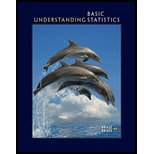# Terminology Consider the following measures of central tendency: mean, median, mode. Match each type to the appropriate description: (i) the central value of a data set after it has been ordered from smallest to largest (ii) the data value occurring most frequently in a data set (iii) the sum of all the data values in a data set divided by the number of data values in the set### Understanding Basic Statistics

8th Edition
Charles Henry Brase + 1 other
Publisher: Cengage Learning
ISBN: 9781337558075### Understanding Basic Statistics

8th Edition
Charles Henry Brase + 1 other
Publisher: Cengage Learning
ISBN: 9781337558075

#### Solutions

Chapter
Section
Chapter 3, Problem 1CR
Textbook Problem

## Terminology Consider the following measures of central tendency: mean, median, mode. Match each type to the appropriate description:(i) the central value of a data set after it has been ordered from smallest to largest(ii) the data value occurring most frequently in a data set(iii) the sum of all the data values in a data set divided by the number of data values in the set

Expert Solution
To determine

The mean or median or mode type to the appropriate description.

Solution: Median for (i), mode for (ii), and mean for (ii).

### Explanation of Solution

The median of a set of data values is the middle (center) value of the data set when it has been arranged in ascending order. To find the median, organize each number in order by size; the number in the middle is the median.

Hence, the (i) description that the central value of a data set after it has been ordered from smallest to largest is called the median of the data.

The mode is the most common number in the set of data or this is the number that occurs most often. The average that represents the most frequent value of the distribution is called mode. So, the mode is appropriate to (ii) description.

The mean is often called the average. To find the mean, take a set of data and calculate the sum of the data, after that divide the sum by the number of pieces in the set. Hence, mean takes all specific values into account. The mean is appropriate to (iii) description.

### Want to see more full solutions like this?

Subscribe now to access step-by-step solutions to millions of textbook problems written by subject matter experts!

Get Solutions

### Want to see more full solutions like this?

Subscribe now to access step-by-step solutions to millions of textbook problems written by subject matter experts!

Get Solutions